# Ratio - 6th grade (11y) - math problems

On solving problems and tasks with proportionally, we recommend hint rule of three. Rule of three (proportionality) help solve examples of direct and inverse proportionality. Three members make possible to calculate the fourth - unknown member.

#### Number of problems found: 120

• Mixing paint with waterMr. Adamek will paint. The purchased paint is diluted with water in a ratio of 1: 1.5. a) how many parts of water will add to 1 part of the paint b) how many liters of water the mission adds to 2 liters of paint
• The angles ratioThe angles in the ABC triangle are in the ratio 1: 2: 3. find the sizes of the angles and determine what kind of a triangle it is.
• Two villages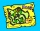On the map with a scale of 1:40000 are drawn two villages actually 16 km away. What is their distance on the map?
• The perimeter 3The perimeter of a rectangle is 35 cm. The ratio of the length to its width is 3:2. Calculate the dimensions of the rectangle
• Geometric plan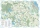At what scale the building plan if one side of the building is 45m long and 12mm long on a plan?
• Barter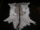There is exchange trade on the market. We know that for two sheepskins we get three goat skins. We also know that for six rabbits skins we get four goat skins. How many rabbit's skins we get for four sheepskins?
• Area of rectangleHow many times will increase the area of the rectangle, if we increase twice the length and at the same time we decrease the width by the half?
• Five mechanicsFive mechanics will make a car for some time. How many cars can 30 mechanics produce at the same time?
• Temperature 5Temperature outside starts at 0 Fahrenheit. Over time the temperature changes at a rate of -0.6 Fahrenheit per hour. How long will it take for the temperature to reach -4.5 Fahrenheit?
• Land area 2A land area was divided among the three heirs in the ratio 5:2:4. If the largest share was 20 hectares of land, what is the total area of land? Please show your solution and what kind of proportion is this please
• Three friendsThree friends had balls in ratio 2: 7: 4 at the start of the game. Could they have the same number of balls at the end of the game? Write 0, if not, or write the minimum number of balls they had together.
• Scale of planOn the plan of the village in the scale of 1: 1000 a rectangular garden is drawn. Its dimensions on the plan are 25mm and 28mm. Determine the area of the garden in ares.
• Two brothersThe two brothers were to be divided according to the will of land at an area of 1ha 86a 30m2 in a ratio of 5:4. How many will everyone get?
• BuildingAt the building, we divided 240 boards into two piles in a 5: 3 ratio. How many were fewer boards in the lower pile?
• AndreAndre, Thomas, and Ivan split 88 postage stamps in a 2:5:4 ratio. How much did Thomas get?
• Babysitting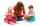The amount that Susan charges per hour for babysitting are directly proportional to the number of children she is watching. She charges \$4.50 for 3 kids. How much would she charge to babysit 5 kids?
• Scale of the map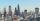The distance between two cities is actually 30 km and the map is 6 cm. What is the scale of the map?
• GardenThe rectangular garden has dimensions of 27 m and 30 m. Peter and Katka split it in a ratio of 4:5. How many square meters did Katkin measure part of the garden?
• PamelaLara Pamela spends her salary of 3000 for food, clothing, recreation and savings, which are in the ratio of 48:20:15:37, respectively. How much does he spend for savings?
• Kilo candyOne kilogram of chocolate candy cost 10 euros. Kate buys 250 grams and had the 8 pack of candy. How much does cost one bonbon?

Do you have an interesting mathematical word problem that you can't solve it? Submit a math problem, and we can try to solve it.

We will send a solution to your e-mail address. Solved examples are also published here. Please enter the e-mail correctly and check whether you don't have a full mailbox.

Please do not submit problems from current active competitions such as Mathematical Olympiad, correspondence seminars etc...

Check out our ratio calculator.